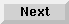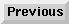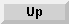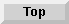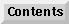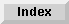10 Matrix Operations

## Assigning Values to Arrays

### MAT INPUT

The MAT INPUT statement suspends program execution, allowing values in the form of expressions to be assigned to elements of arrays from the keyboard. Syntax for this statement is as follows:

MAT INPUT array1 [(redim subscripts)] [,array2] [(redim subscripts)]

When MAT INPUT is executed, a question mark (?) appears in the display line. Values in the form of numeric expressions can be assigned individually or in groups (separate each value with a comma). Values are stored by pressing RETURN. The ? is redisplayed after RETURN is pressed until all values are input.

Pressing RETURN without entering a value causes execution to continue with the next element in the array. Elements not assigned values retain their previous value. For example:

```10   OPTION BASE 1
20   INTEGER A(2,2)
30   MAT INPUT A
```
Responding to the MAT INPUT statement by typing 2, typing 4, pressing RETURN, and typing 8, assigns the following values:

```A(1,1)=2
A(1,2)=4
A(2,1)=0    Note that the initial value, 0, is kept
A(2,2)=8
```
The following statements are equivalent:

```MAT INPUT A                     INPUT A(*)
```
The MAT INPUT statement is programmable only; it cannot be executed from the keyboard.

### MAT . . . CON

Use the MAT . . . CON statement to assign the constant 1 to all elements of an array. Syntax for this statement is as follows:

MAT array = CON [(redim subscripts)]

Since 1 has a logical value of "true", the constant matrix is useful for logical initialization.

The following two sequences are equivalent:

```10   DINTEGER A(10,10)           10   OPTION BASE 1
20   MAT A=CON                   20   DINTEGER A(10,10)
30   FOR I=1 TO 10
40     FOR J=1 TO 10
50       A(I,J)=1
60     NEXT I
70   NEXT J
```

### MATZER

Use the MATZER statement to assign the value 0 to all elements of an array. Syntax for this statement is as follows:

MAT array = ZER [(redim subscripts)]

Since 0 has a logical value of "false", the zero matrix is useful for logic initialization.

The following two sequences are equivalent:

```10  REAL A(12,12,12)             10   REAL A(12,12,12)
20  MAT A=ZER                    20   FOR I=1 TO 12
30     FOR J=1 TO 12
40       FOR K=1 TO 12
50         A(I,J,K)=0
60       NEXT K
70     NEXT J
80   NEXT I
```

### MAT-initialize

The MAT-initialize statement assigns the same value to every element in an array. Syntax for this statement is as follows:

MAT array = (numeric expression or string expression)

The numeric expression is evaluated once; it is converted to the numeric type of the array, if necessary. For example:

```150   INTEGER X(4,4)                    150   DIM A\$(4)
160   MAT X=(PI)                        160   MAT A\$=("TEST")
170   MAT PRINT X;                      170   MAT PRINT A\$;
3  3  3  3                                 TEST
3  3  3  3                                 TEST
3  3  3  3                                 TEST
3  3  3  3                                 TEST
```

Eloquence Language Manual - 19 DEC 2002